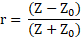# The Basics of Time Domain Reflectometry (TDR)Cable Fault Location

The time domain reflectometry (TDR) method is the most established and widely used measuring method for the determination of:

• the total length of a cable
• the location of low resistive cable faults
• the location of cable interruptions
• the location of joints along the cable

A TDR sends a low voltage pulse into the cable under test and at any impedance change within the cable a reflection will be seen. The TDR measures the time between release and return of the low voltage pulse from any reflections. By measuring the time and knowing the propagation velocity of the pulse, the distance to the reflection can be calculated. This helps to give a user information on the type of impedance change or failure that could be present in the cable.

The below image shows a typical set-up for a TDR measurement. A parallel path of two conductors is required to see a reflection graph of a pulse traveling along a cable. Therefore, one of the leads of the TDR is connected to one phase of the cable and the other lead is connected to the cable sheath, which is also connected to ground. The reflection of the pulse is caused by the change of impedance between those two paths and every interruption, change of impedance, or cable end is indicated. The low voltage pulse travels through the cable and is reflected positively at the cable end or at any cable interruption (cable cut). At a short circuit point this low voltage impulse is reflected negatively. A change of the impedance in the cable, such as with a joint, will be displayed as a laid S.Below are a few more examples of typical impedance changes that occur in cables and can be seen using TDRs:The magnitudes of the pulse reflections are determined by the reflection coefficient:where r is the reflection coefficient, Z is the impedance value of the deviation from the homogenous structure of the cable (i.e., cable start, joints, faults, changes in cable type, cable end, etc.), and Z0 is the cable’s characteristic impedance.

Hence, for an open end Z >> Z0 and r is a positive reflection, whereas with a short circuit Z << Z0 and r is a negative reflection.

Determining the exact distance of a reflection is dependent upon the time the pulse takes to travel from the TDR and back along a certain length and also on the propagation velocity of the pulse. The formula for determining this is:

l = t x v/2, where l is length, t is time and v is velocity.

The TDR instrument measures the time it takes for the pulse to return. If the exact length of cable is known, then the formula can be used to determine the propagation velocity. If the length is not known, and this is the case most times, then if the propagation velocity is known, the length can be calculated.

Many times, a user may not know the length of cable or propagation velocity. The velocity is dependent upon the dielectric or insulation between the two conductors and also on the condition of the cable, such as corrosion, water ingress, etc. In these cases, it helps to use rule of thumb propagation velocities, depending on the type of cable being tested.

Type of Cable Typical Propagation Velocity
XLPE 82 – 86 m/µs (269 – 282 ft/µs)
PILC 77 – 82 m/µs (253 – 269 ft/µs)
Hybrid 83 m/µs (272 ft/µs)
Overhead wire 148 m/µs (485 ft/µs)
Telecommunication 95 – 120 m/µs (312 – 394 ft/µs)

Check out our handheld TDR 500 or TDR 510